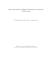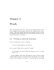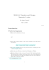# PROBLEM SET 01 - Proofs and induction 1 Proofs 2 Induction

## Transcription

PROBLEM SET 01 - Proofs and induction 1 Proofs 2 Induction
```PROBLEM SET 01 - Proofs and induction
1
Proofs
Exercise 1.1. Give a direct proof that if m and n are both perfect squares, then nm is also a perfect square. (An integer
a is a perfect square if there is an integer b such that a = b2 .)
Exercise 1.2. Prove that the sum of two rational numbers is rational. (Note that if we include the implicit quantifiers
here, the theorem we want to prove is "For every real number r and every real number s, if r and s are rational numbers,
then r + s is rational.)
Exercise 1.3. Prove by
√ contradiction that if n > 1 and n is non prime natural number then it has at last one prime
divisor p such that p ¬ n.
Exercise 1.4. Prove that if m + n and n + p are even integers, where m, n, and p are integers, then m + p is even.
Exercise 1.5. Show that if n is an integer and n3 + 5 is odd, then n is even using
a) a proof by contraposition.
Exercise 1.6. Show that if you pick three socks from a drawer containing just blue socks and black socks, you must get
either a pair of blue socks or a pair of black socks.
Exercise 1.7. Prove or disprove the statement: Every positive integer can be written as the sum of the squares of three
integers.
Exercise 1.8. Prove that at least one of the real numbers a1 , a2 , . . . , an is greater than or equal to the average of these
numbers. What kind of proof did you use?
2
Induction
Basic induction
Exercise 2.1. Prove that for all n ­ 1
7 | 23n−1 + 3.
Exercise 2.2. Prove that for all n ­ 1
12 + 22 + 32 + . . . + n2 =
n(n + 1)(2n + 1)
.
6
Exercise 2.3. Prove that for all n ­ 3
nn+1 > (n + 1)n .
Strong induction
Exercise 2.4. Show that if n is an integer greater than 1, then n can we written as a product of primes.
Exercise 2.5. Define a sequence (cn ):


c0 = −3;
c1 = 1;


cn = 2cn−1 − cn−2
for n ­ 2.
Prove that cn = 4n − 3 for n ­ 0.
Exercise 2.6. Consider a game in which two players take turns removing any positive number of matches they want from
one of two piles of matches. The player who removes the last match wins the game. Show that if the two piles contain
the same number of matches initially, the second player can always guarantee a win.
Exercise 2.7. Prove that every amount of postage of 12 cents or more can be formed using just 4-cent and 5-cent stamps.
Exercise 2.8. Assume that a chocolate bar consists of n squares arranged in a rectangular pattern. The entire bar, a
smaller rectangular piece of the bar, can be broken along a vertical or a horizontal line separating the squares. Assuming
that only one piece can be broken at a time, determine how many breaks you must successively make to break the bar
Exercise 2.9. Consider this variation of the game of Nim. The game begins with n matches. Two players take turns
removing matches, one, two, or three at a time. The player removing the last match loses. Use strong induction to show
that if each player plays the best strategy possible, the first player wins if n = 4j, 4j +2, or n = 4j +3 for some nonnegative
integer j and the second player wins in the remaining case when n = 4j + 1 for some nonnegative integer j .
1
```

### Assignment 2 - Technische Universiteit Eindhoven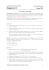### Sample Final Exam Questions (Mandatory Part)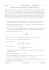### Geometry 4.7 worksheet Name Period _____ 1. Sketch the### Sample Problems = +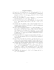### Sample Problems Algebra### Sample Problems Math 334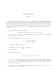### Discrete Math, Spring 2013 - Some Sample Problems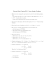### Adding auxiliary lines: Student worksheet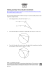### 2015 Exam - MAA Sections - Mathematical Association of America